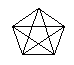Email us to get an instant 20% discount on highly effective K-12 Math & English kwizNET Programs!

#### Online Quiz (WorksheetABCD)

Questions Per Quiz = 2 4 6 8 10

### Grade 5 - Mathematics7.13 Diagonals

 A diagonal is a line segment that is not a side and that joins two vertices of a polygon. Directions: Answer the following. Also draw a triangle, square and rectangle and show its diagonals.
 Q 1: A diagonal is a line segment that is not a side and that joins two vertices of a polygon.falsetrue Q 2: The figure gives the number of sides and their diagonals. What is the pattern?2,4,5,61,2,3,43,4,5,6,7 Q 3: A pentagon has ______diagonals.5467 Q 4: A quadrilateral has ___ diagonals.234 Q 5: From the figure can you find out the number of diagonals for a 10 sided polygon?372735 Q 6: If the diameter of a circle is 28 meters, what is the radius?85 meters28 meters14 meters2 meters Question 7: This question is available to subscribers only! Question 8: This question is available to subscribers only!